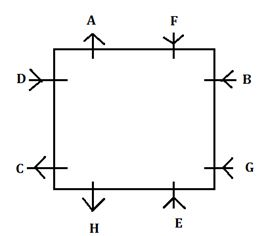Latest Banking jobs   »   Reasoning Ability Quiz For IBPS Clerk...

# Reasoning Ability Quiz For IBPS Clerk Prelims 2021- 8th August

Directions (1-5): Study the following information carefully to answer the given questions.
A B, C, D, E F, G and H are sitting around a Square table such that two persons sit on each side, but not necessarily in the same order. Some of the people are facing the centre while some face outside. C sits second to the left of E. E faces the centre. F sits third to the right of C. G sits second to the left of H. G faces the same direction as D. Immediate neighbours of E and F face opposite directions (i.e., if one neighbour faces the centre the other neighbour faces outside and vice-versa). H doesn’t face towards the center. G sits opposite to C. D sits third to the right of B. E sits second to the left of B. Immediate neighbours of B face the same direction (i.e., if one neighbour faces the centre the other neighbour also faces the centre and vice-versa)

Q1. How many people are sitting between G and C when counted in clockwise direction from G?
(a) Two
(b) Three
(c) One
(d) Four
(e) Five

Q2. Which of the following is TRUE regarding E as per the given seating arrangement?
(a) H sits second to the left of E
(b) E faces inside
(c) Only two people sit between E and F when counted in clockwise direction from E
(d) C is one of the immediate neighbors of E
(e) Only three people sit between E and G

Q3. Four of the following five are alike in a certain way based on the given seating arrangement and so form a group. Which is the one that does not belong to that group?
(a) C
(b) A
(c) H
(d) D
(e) None of these

Q4. What is B’s position with respect to A?
(a)Third to the left
(b)To the immediate left
(c)To the immediate right
(d)Second to the right
(e)Third to the right

Q5. Who sits second to the left of D?
(a) H
(b) A
(c) B
(d) Other than those given as options
(e) F

Directions (6-10): The following questions are based on the five three digits numbers given below:
135 241 569 748 873

Q6. If in each number, all the three digits are arranged in ascending order within the number, which of the following will be the second lowest number after rearrangement?
(a) 873
(b) 748
(c) None of these
(d) 569
(e) 135

Q7. If second digit will be interchanged with third digit in each number, then which number will be the second highest number after rearrangement?
(a) 873
(b) 748
(c) None of these
(d) 569
(e) 135

Q8. If in each number, both first and third digits are added and after that second digit is subtracted from that addition then how many numbers are greater than 5 after mentioned operation?
(a) One
(b) Two
(c) Three
(d) Four
(e) None of these

Q9. If in each number, all the odd digit is subtracted by 1 and all even digit is added by 1 then, which of the following will be the lowest number after mentioned operation?
(a) 873
(b) 748
(c) None of these
(d) 569
(e) 135

Q10. If 1 is subtracted from first digit and 2 is added in last digit of each number then which number will be the 4th lowest number after mentioned operation?
(a) 873
(b) 748
(c) None of these
(d) 569
(e) 135

Directions (11-15): In these questions, relationship between different elements is shown in the statements. These statements are followed by two conclusions.
(a) If only conclusion I follows.
(b) If only conclusion II follows.
(c) If either conclusion I or II follows.
(d) If neither conclusion I nor II follows.
(e) If both conclusions I and II follow.

Q11. Statements: A≤M>G >K >D, M≤O< S, K<H
Conclusions:
I. G < S
II. A > D

Q12. Statements: B ≥ E >L<O>R=U
Conclusions:
I. B<L
II. U=L

Q13. Statements: X ≥ Q > F, A > F, R = M ≥ F
Conclusions:
I. R > A
II. X > F

Q14. Statements: D ≤ G > K ≥ R > T, G ≤ E > W, R < Y
Conclusions:
I. W ≥ D
II. Y > T

Q15. Statements: B ≥ K > G, M ≤ G, D = W ≥ G
Conclusions:
I. D ≥ M
II. K < W

Solutions

Solutions (1-5):
Sol.S1. Ans. (a)
S2. Ans. (b)
S3. Ans. (d)
S4. Ans. (d)
S5. Ans. (e)

S6. Ans.(e)
Sol. 135, 124, 569, 478, 378

S7. Ans.(b)
Sol. 153 214 596 784 837

S8. Ans.(b)

S9. Ans.(e)

S10. Ans.(b)

S11. Ans.(a)
Sol. I. G < S (true) II. A > D (false)

S12. Ans.(d)
Sol. I. B<L (false) II. U=L (false)

S13. Ans.(b)
Sol. I. R > A (false) II. X > F (true)

S14. Ans.(b)
Sol. I. W ≥ D (false) II. Y > T (true)

S15. Ans.(a)
Sol. I. D ≥ M (true) II. K < W (false)#### Congratulations!Union Budget 2023-24: Free PDF